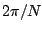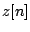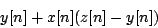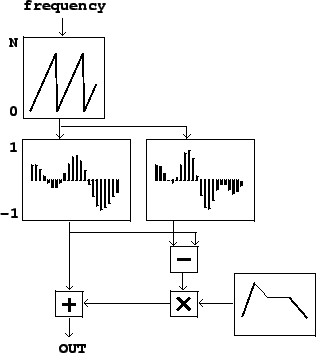Next: Sampling Up: Wavetables and samplers Previous: Wavetables and samplers   Contents   Index

# The Wavetable Oscillator

Figure 2.2 suggests an easy way to synthesize any desired fixed waveform at any desired frequency, using the block diagram shown in Figure 2.3. The upper block is an oscillator--not the sinusoidal oscillator we saw earlier, but one that produces sawtooth waves instead. Its output values, as indicated at the left of the block, should range fromto the wavetable size. This is used as an index into the wavetable lookup block (introduced in Figure 2.1), resulting in a periodic waveform. Figure 2.3 (part b) adds an envelope generator and a multiplier to control the output amplitude in the same way as for the sinusoidal oscillator shown in Figure 1.7 (Page). Often, one uses a wavetable with (RMS or peak) amplitude 1, so that the amplitude of the output is just the magnitude of the envelope generator's output.Wavetable oscillators are often used to synthesize sounds with specified, static spectra. To do this, you can pre-computesamples of any waveform of period(angular frequency) by adding up the elements of the Fourier Series (Page). The computation involved in setting up the wavetable at first might be significant, but this may be done in advance of the synthesis process, which might take place in real time.

While direct additive synthesis of complex waveforms, as shown in Chapter 1, is in principle infinitely flexible as a technique for producing time-varying timbres, wavetable synthesis is much less expensive in terms of computation but requires switching wavetables to change the timbre. An intermediate technique, more flexible and expensive than simple wavetable synthesis but less flexible and less expensive than additive synthesis, is to create time-varying mixtures between a small number of fixed wavetables. If the number of wavetables is only two, this is in effect a cross-fade between the two waveforms, as diagrammed in Figure 2.4. Suppose we wish to use some signalto control the relative strengths of the two waveforms, so that, if, we get the first one and ifwe get the second. Denoting the two signals to be cross-faded byand, we compute the signalor, equivalently and usually more efficient to calculate,This computation is diagrammed in Figure 2.4.When using this technique to cross-fade between wavetable oscillators, it might be desirable to keep the phases of corresponding partials the same across the wavetables, so that their amplitudes combine additively when they are mixed. On the other hand, if arbitrary wavetables are used (borrowed, for instance, from a recorded sound) there will be a phasing effect as the different waveforms are mixed.

This scheme can be extended in a daisy chain to move along a continuous path between a succession of timbres. Alternatively, or in combination with daisy-chaining, cross-fading may be used to interpolate between two different timbres, for example as a function of musical dynamic. To do this you would prepare two or even several waveforms of a single synthetic voice played at different dynamics, and interpolate between successive ones as a function of the output dynamic you want.Next: Sampling Up: Wavetables and samplers Previous: Wavetables and samplers   Contents   Index
Miller Puckette 2006-12-30